# HSPT Quantitative : How to make non-geometric comparisons

## Example Questions

### Example Question #61 : Non Geometric Comparison

Examine (a), (b), and (c) to find the best answer:

a)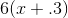b)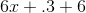c)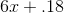(a) equals (b) but not (c)

(a) equals (c) but not (b)

(a), (b), and (c) are all unequal

(a), (b), and (c) are all equal

(a) equals (c) but not (b)

Explanation:

To compare the expressions, distribute and simplify:

a)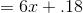b)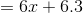c)It is now clear that (a) and (c) are equal but (b) is not.

### Example Question #61 : How To Make Non Geometric Comparisons

Examine (a), (b), and (c) to find the best answer:

a)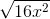b)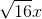c)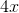(a) is equal to (b) but not (c)

(a) is equal to (c) but not (b)

(a), (b), and (c) are all unequal

(a), (b), and (c) are all equal

(a), (b), and (c) are all equal

Explanation:

The important property of square roots to remember here is that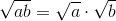This means that (a) can be broken up into the following: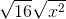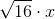These variations are all equal

### Example Question #63 : Non Geometric Comparison

Examine (a), (b), and (c) to find the best answer:

a)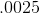b)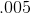c)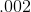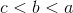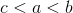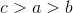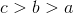Explanation:

(a)is exactly half of (b), and is therefore smaller. (c)is even smaller than both.

### Example Question #64 : Non Geometric Comparison

Examine (a), (b), and (c) to find the best answer:

a)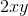b)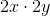c)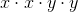(a) is equal to (b) but not (c)

(a), (b), and (c) are all equal

(a) is equal to (c) but not (b)

(a), (b), and (c) are all unequal

(a), (b), and (c) are all unequal

Explanation:

One way to test this answer is to substitute in numbers for the variables. For example, let's say thatand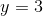:

a)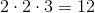b)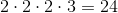c)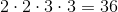None are equal!

### Example Question #65 : Non Geometric Comparison

Examine (a), (b), and (c) to find the best answer:

a)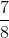ofb)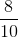ofc)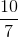of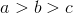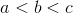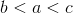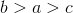Explanation:

Multiply the fractions by the integers in order to compare the expressions:

a)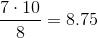b)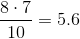c)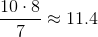It is now clear that (b) is smaller than (a), which is smaller than (c).

### Example Question #66 : Non Geometric Comparison

Examine (a), (b), and (c) to find the best answer:

a)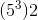b)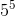c)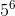(a), (b), and (c) are all unequal

(a) equals (c) and is less than (b)

(a), (b), and (c) are all equal

(a) equals (c) and is greater than (b)

(a) equals (c) and is greater than (b)

Explanation:

The property of exponents to remember here is that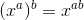.

This means that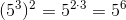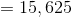.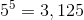.

### Example Question #67 : Non Geometric Comparison

Examine (a), (b), and (c) to find the best answer:

(a)(b)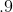(c)percent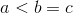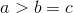Explanation:

Converted into decimals, the numbers look like this:

a)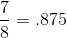b)c)percent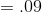It is now evident that (c) is smaller than (a), which is smaller than (b)

### Example Question #68 : Non Geometric Comparison

Examine (a), (b), and (c) to find the best answer:

a)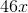b)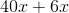c)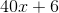(a) equals (b) but not (c)

(a), (b), and (c) are all equal

(a), (b), and (c) are all unequal

(a) equals (c) but not (b)

(a) equals (b) but not (c)

Explanation:

Try substituting a number in forto test this problem. Here, we try.

a)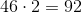b)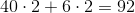c)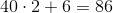It is now evident that (a) and (b) are equal, but not (c)

### Example Question #61 : How To Make Non Geometric Comparisons

Examine (a), (b), and (c) to find the best answer:

a)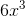b)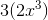c)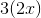(a) is equal to (b) but not (c)

(a) is equal to (c) but not (b)

(a), (b), and (c) are all unequal

(a), (b), and (c) are all equal

(a) is equal to (b) but not (c)

Explanation:

(b) is a factored version of (a). (c), however, is not raised to the same exponent. Test this by plugging a number in for. Here, we try.

a)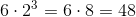b)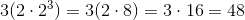c)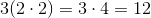(a) and (b) are equal, but (c) is not.

### Example Question #70 : Non Geometric Comparison

Examine (a), (b), and (c) to find the best answer:

a)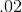percent

b)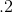c)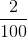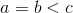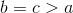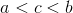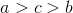Explanation:

Convert each to a decimal in order to compare them:

a)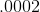b)c)It is now evident that (a) is smaller than (c), which is smaller than (b).